Digging Deeper into … Number Sentences, Equations and Variables (3rd – 6th)

Digging Deeper into … Number Sentences, Equations and Variables (3rd – 6th)

In the Primary Mathematics Curriculum (1999), this topic appears as three separate strand units, all within the strand of Algebra:

• Number Sentences (3rd & 4th class)
• Equations (5th & 6th class)
• Variables (6th class)

However, since these concepts are intrinsically connected, in Operation Maths they are taught in a cohesive and progressive way through third to sixth class.

• Number sentences are mathematical sentences written using numerals (e.g. 1, 5, 67, 809, 1.45, 1/2  etc.) and mathematical symbols (e.g. +, -, x, ÷, <, >, =).
• They include both equations (see below) and inequalities (64 < 82, 23 > -16), although the term inequalities is not specifically used.
• The unknown or missing value in a number sentence (i.e. a variable) can be represented by a frame (box), by a shape, or by a letter, although it should be noted that the Primary Mathematics Curriculum (1999) specifies a preference for a frame (box), up to the introduction of variables in 6th class
• An Equation is a special type of number sentence, containing an equals sign, to show that two expressions are equal (e.g. 5 = 3 + 2, 5 + 6 = 20 – 9, etc.)
• A variable is a value in an expression that can  change or vary. However, when there is only one variable in an equation then the value of that variable can be calculated e.g. a + 6 = 9, 20 = 4b.

Thus, while these strand units are only being formally introduced from third class on, the children have actually been exposed to number sentences, equations and variables (i.e. the frame) since the infant classes.

Equations

(aka Number sentences with an equals sign)

Understanding equations necessitate the appreciation of the correct meaning of the equals symbol. Many children incorrectly translate the equals symbol (=) as meaning ‘and the answer is…’. This incorrectly reinforces that both its purpose and position is to precede the answer in any calculation, a misconception also reinforced by calculators, where you press the = button to get the answer. Such misunderstanding is
evident when you see responses like these:
5 + 6 =  + 3 , i.e. ‘5 + 6 is 11’
5 + 6 =  + 3 , i.e. ‘5 + 6 + 3 is 14’
Adults may also unwittingly compound this, by using ‘makes’ or ‘gives’ as a synonym for equals.

It is vital that the children recognise that the equals symbol indicates that both sides of the equation (which will be referred to simply as a number sentence until fifth class, when the term “equation” is introduced), are equal to one another/are the same value/are balanced. In this way an actual balance (pan or bucket) and cubes can be extremely valuable to model (and solve) equations e.g. in the images below, the first balance shows that 5 equals a group of 3 and a group of 2, and the second balance shows that 12 equals 3 groups of 4.From Operation Maths 4From Operation Maths 5

Furthermore, teachers should reinforce the correct meaning for the symbol = by only translating it as ‘equals’, ‘is equal to’ and/or ‘is the same as’.

Inequalities

(aka Number sentences with greater than/less than sign)

Despite the fact that the children have been using the greater than and less than symbols since 2nd Class, many still have difficulties reading them and interpreting their meaning. Using a balance and concrete materials, in a similar way as when teaching equations, can greatly help children to gain deeper understanding of the symbols and their meanings.From Operation Maths 4

Through exploration they can identify what is the maximum number of cubes they can put on a side that is less than the other side, before it makes the balance tip in the other direction, thereby invalidating the number sentence; or the minimum number of cubes they can put on a side that is greater than the opposite side, so as to keep the number sentence true.

Using estimation strategies

Often, when having to indicate if a given number sentence is true or false, it is not always necessary for the children to calculate both sides of the number sentence exactly. There is (usually) only one true or correct option, meaning that every other answer is incorrect or false. Encourage the children to use their estimation and number sense skills to quickly recognise when a statement is obviously false, e.g. a big difference in the size of numbers on one side versus the other.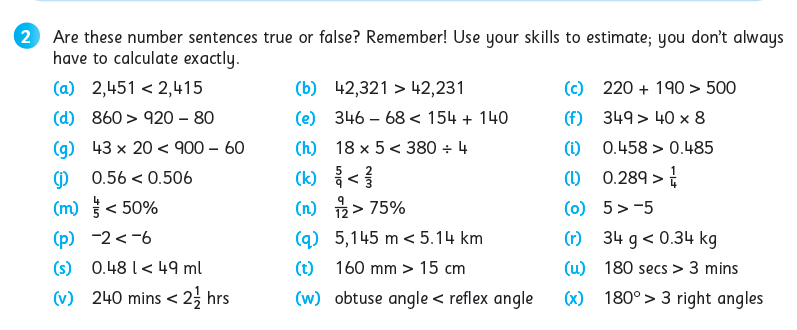While some might view this as a type of ‘cheat’ strategy, in truth, it is more about identifying the most efficient approach, while also reinforcing the value of estimation in general and, particularly, as a way to make calculations easier.

Translating number sentences into word problems and vice-versa

As mentioned earlier, this is in fact a skill that the children would have been exposed to, and been using, since the infant classes. Furthermore, as this topic is deliberately positioned towards the end of the yearly plans in Operation Maths 3-6, the children will have already been using this skill very regularly in the number, data and measures chapters, prior to this point of the school year.

The curriculum specifies that the children should be enabled to translate number sentences into word problems, both of which can be viewed as abstract representations. Worth noting, is that the curriculum doesn’t emphasise the importance of the translating the number sentences and word problems into concrete and/or pictorial representations. Whereas, in Operation Maths, (in keeping with its overarching CPA approach) , there is significant emphasis placed also on utilising various concrete materials and visual strategies to represent the word problems and number sentences.From Operation Maths 5

The development of visual strategies for problem-solving,  is a central focus of the work throughout the Number chapters. Thus, this topic allows the teacher to revise the visual strategies covered so far and assess how well the children understand them and can apply them.

The interconnectedness of real-life scenarios and mathematical sentences/equations should also be emphasised. At primary level, there should always be some relatable context for any number sentence.
For many children, when looking at a number sentence, it can be difficult to appreciate how a collection of digits and symbols could relate to a real-life scenario with which they can identify. That is essentially what a word problem is; it provides a real-life context within which to frame the numbers and operators involved. Emphasise to the children throughout this topic how the number sentences could be given a real-life story (i.e. word problem), and encourage them to come up with possible stories either verbally or written down.

And, depending on the context given to a particular story, the visual representation may also be different, even though the number sentence/equation may stay the same. For example for the number sentence 7 – 4 = ? the word problem (context) could be either of the following:

• Áine has 7 cookies. Abdul has 4 cookies. How many more cookies has Áine than Abdul?Image created using Bar Modelling eManipulative, accessible on edcolearning.ie

And while the number sentences are the same, both the contexts and the pictorial representations (e.g. bar models, as shown above) are different, as they represent different types of subtraction. In the case of the first word problem, this is describing subtraction as deduction, and a part-whole bar model is more suitable. In the case of the second word problem, this is describing subtraction as difference, and a comparison bar model is more suitable.

Identifying operation phrases

When the children are translating word problems into number sentences, it is very important that they can understand the context being described and are able to identify that phrases that indicate the operation(s) required.

Regularly interspersed throughout the operations chapters in the Discovery books for Operation Maths 3-6,  there are activities which enable the children to identify and colour-code the specific vocabulary that an indicate the required operation (see example below). This topic provides an ideal opportunity to review this skill and assess/re-teach the children accordingly.From Operation Maths 4, Discovery Book

In particular, many of the Talk Time activities, require the children to suggest ways to verbalise the various equations, e.g:

• The difference between 46 and 18 is equal to the product of 4 and 7; true or false?
• 18 subtracted from 46 equals 4 times 7; true or false?

Where possible the children should suggest alternative phrases for the same equation thus reinforcing the use of correct mathematical language.

Input and Outputs

In Operation Maths 4 & 5, activities based on inputs and outputs are included as a means to consolidate the children’s understanding of number sentences and their ability to write number sentences (see below).  Input-output activities can provide great scope for problem solving, as well as preparing the children for calculations involving variables in sixth class.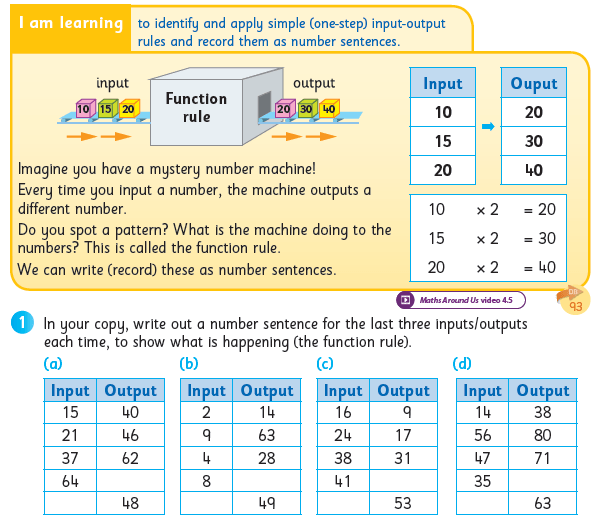From Operation Maths 4

Variables

Variables are formally introduced in sixth class, although the children have encountered variables (as a symbol or shape to represent a missing value) since they first encountered the frame (answer box).

When calculating with variables, both part-whole bar models and comparison bar models can be very useful to represent the relationship between the known and unknown values.From Operation Maths 6

Digging Deeper into … Directed Numbers (5th & 6th classes)

Category : Uncategorized

For practical suggestions for families, and links to useful digital resources, to support children learning about the topic of area, please check out the following post: Dear Family, your Operation Maths Guide to Directed Numbers

In Operation Maths for 5th Class the children are formally introduced to the concept of directed numbers (from the strand of algebra) and are enabled to:

• Explore and identify directed numbers, i.e. numbers above and below zero, including zero
• Describe and record directed numbers as positive or negative
• Use directed numbers to represent real-life situations
• Compare and order directed numbers
• Solve problems involving directed numbers.

In Operation Maths for 6th class, this knowledge and understanding is revised and extended to include the addition of positive and negative numbers.

Directed numbers (formally known as integers, the set of positive and negative whole numbers including zero) can be a very difficult concept for some children for a number of reasons:

• Being able to explore numbers less than zero requires a solid understanding of zero, an abstract concept in itself since it indicates the absence of something
• Many children find it difficult to comprehend how something can be less than zero. Therefore, when ordering it can be difficult for many children to appreciate that 0 is greater than -1, -2, etc.
• Operations involving integers are particularly troublesome as having similar signs so close together can be very confusing.

Use real-life contexts

In fifth class, since this is the first time that the children have been formally introduced to negative numbers, it is essential that this happens through reference to real-life contexts, e.g. temperature, buttons in a lift, goal difference in soccer league tables, depths in a swimming pool, or any other context with which the children can readily identify. Other real-life contexts such as bank account balances, elevations, etc., can also be used.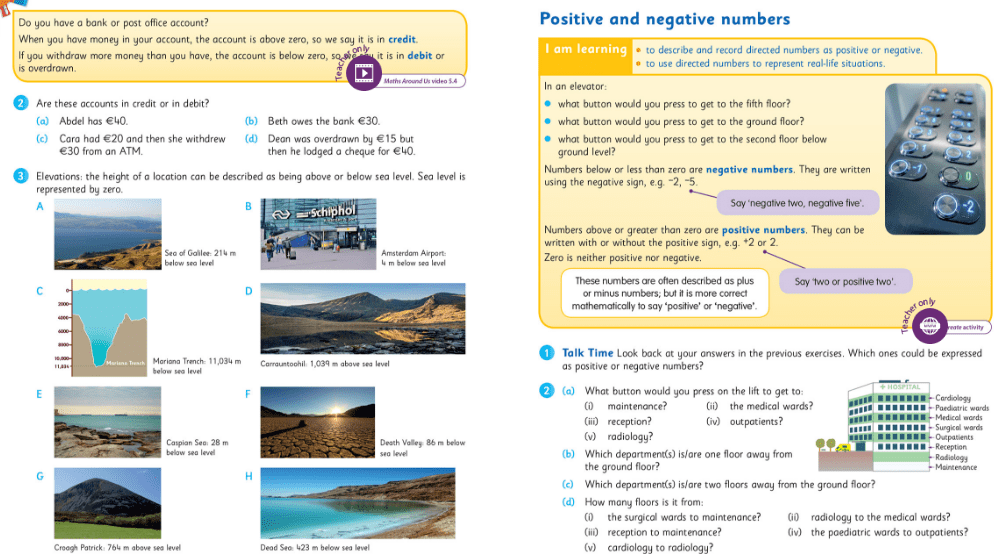Use ‘positive’ and ‘negative’ rather than ‘plus’ and ‘minus’

Whilst real-life contexts are useful, they can also be confusing, particularly when plus and minus are used to describe directed numbers. Insist that the children use the language of positive and negative, since that is more correct mathematically. Using positive and negative can also reduce complications in future classes when calculating with directed numbers, e.g. avoiding having to say three minus minus five for 3-(-5). This is particularly important when the children progress to adding positive and negative numbers: for example (–3) + (+9) should be read as ‘negative three add/plus positive nine’ rather than ‘minus 3 plus plus 9’. It is also important that the children recognise that positive numbers can be written either with, or without, the positive sign, therefore we can assume that any number without a sign is positive.

To further reduce complications with written forms of directed numbers, raised signs should be used for the positive and negative (as shown in image below) and brackets should be used to make it easier for the child to distinguish between the operations signs (i.e. + and -) and the directed numbers signs.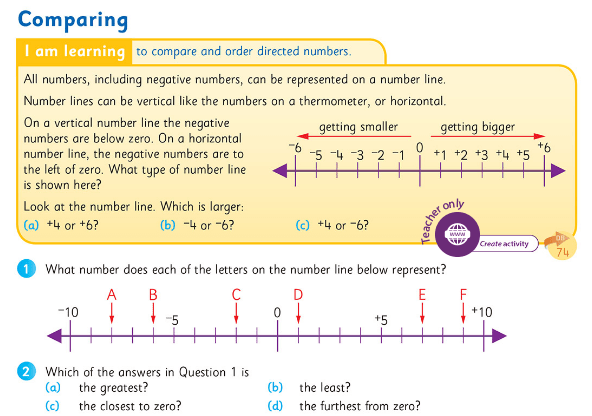Representing directed numbers

Since negative numbers are so abstract, it is vital that opportunities to represent them concretely and pictorially are maximised. Many children consider only the cardinality of numbers, i.e. that they represent the number of objects in a set, whereas understanding of directed numbers relies on understanding the ordinality of numbers; this is why visual representations are so vital.

One way to do this, is to use a counting stick, if available. Start with the counting stick in a vertical position, asking the children that if the centre line is now representing zero, can they identify the points above and below that?

• Initially describe the positions as “one above zero”, “two below zero” etc
• Then, when appropriate, introduce positions as “positive three”, “negative four” etc
• Development: if each interval now represents two/ten/five as opposed to one, how does this change the identity of the positions?
• Finally, move the counting stick into a horizontal position, so that the negative numbers are now to the left of the centre/zero and the positive to the right (as the children see it).

Operation Maths users can also use the counting stick eManipulative to model the counting stick in a horizontal position, as shown below (Hint: zoom the screen in to 150% to view the numbers better).Number lines are also very useful. When using number lines (or a counting stick), emphasize that numbers get smaller in value as you move above/to the left of zero, and larger as you move below/to the right of zero . If possible, have a number line that includes negative numbers on permanent display in the classroom.

The Operation Maths MWBs can also be used to create a dynamic number line for ordering activities. For example, ask individual children to write a specific integer on their MWB. Then ask a group of children, each with different integers,  to stand out and put their MWBs in order. These numbers could even be hung from a ‘washing line’ using clothes pegs.

In sixth class, to better illustrate the processes involved with the addition of integers, teachers are encouraged to use the positive and negative chips, that accompany the sixth class books as part of the free ancillary resources (they’re on the same sheet as the images of the base ten block for modelling decimal numbers).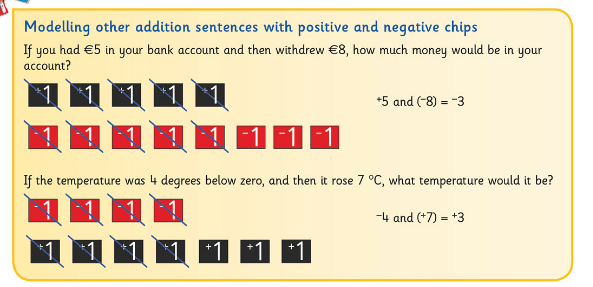The teacher can also use the positive and negative chips built into the Sorting eManipulative to model calculations on the class IWB, as shown below (the positive and negative chips are located at the end of the numbers tab).The children can then progress to using number lines to both reinforce their understanding of addition with integers and extend it to bigger numbers. A physical number line that the children can walk along would be ideal initially, as this suits the kinaesthetic learners and the bigger nature makes it easier for teachers to assess the children’s ability to use the number line before they move to smaller scale number lines in books and copies.• Dear Family, your Operation Maths Guide to Directed Numbers includes practical suggestions for supporting children, and links to a huge suite of digital resources.
• Virtual Maths Manipulatives for Algebra: Lots of tools that can be used in many different ways to explore the Algebra concepts, including directed numbers.
• Operation Maths users don’t forget to use the Counting Stick eManipulative as mentioned earlier and to check out the Maths Around Us video for this topic on Edco Learning. Check out the first page of the Directed Numbers chapter in the Pupils Books for a quick synopsis of the suggested digital resources and then refer to the Directed Numbers chapter in the TRB for more detailed information.
• NRICH: selection of problems, articles and games for negative numbers. In particular, check out Number Lines in Disguise.
• Check out this Pinterest Board of Algebra ideas

Digging Deeper into … Patterns and Sequences

This can be a difficult strand unit to track through the 1999 Primary Mathematics Curriculum; in junior and senior infants it is titled Extending patterns, in first and second classes it becomes Extending and using patterns, in third and fourth it is called Number patterns and sequences,  and in fifth and sixth classes it morphs into Rules and properties. However, it is always from the strand Algebra (check out the maths curriculum glance cards here for more detail) and a summary of the objectives reveals how pattern is at the heart of the strand unit at every class level:

Junior  Infants to Second Class > Algebra > Extending patterns >

• identify, copy and extend patterns (colour, shape, size, number)
• recognise patterns (including odd and even numbers;  predict subsequent numbers)
• explore and use patterns in addition facts (1st & 2nd)

Third & Fourth Class > Algebra > Number patterns and sequences >
• explore, recognise and record patterns in number
• explore, extend and describe (explain rule for) sequences
• use patterns as an aid in the memorisation of number facts

Fifth & Sixth Class > Algebra > Rules and properties >
• identify relationships and record verbal and simple symbolic rules for number patterns

Different types of patterns

So then, are patterns and sequences the same thing? Actually, there are two main types of patterns:

• Repeating patterns: repetitions of symbols, shapes, numbers etc., that recur in a specific way.
• Increasing (growing) and decreasing (shrinking) patterns: An ordered set of shapes or numbers that are arranged according to a rule. Typically, sequence is also used to describe an increasing or decreasing pattern, particularly if it is a pattern of numbers.

Repeating Patterns

A repeating pattern should have a clearly identifiable core, i.e. the shortest sequence that repeats. It is a good idea to use the terminology of “core” right from the infant classes so that the children understand what is being asked of them.Children can often copy patterns without even recognising or identifying the core. However, to become competent in accurately extending repeating patterns, it is vital to identify the core. Ways that the children can become more adept at this include verbalising the pattern out loud (“red, blue, yellow, red, blue, yellow, …”) and/or using concrete materials to model the pattern (see below); in this way it is easier to identify the core of the pattern by breaking it apart and laying it alongside the subsequent parts of the pattern to ensure that they match. This strategy of breaking and matching can also be used to help children check have they extended the pattern correctly. It is also for this reason that cubes and links can often be the easiest concrete materials to use for replicating and extending patterns and are preferable initially to threading beads, pegs on a pegboard etc . These can be used instead when the children are ready to progress to more challenging tasks.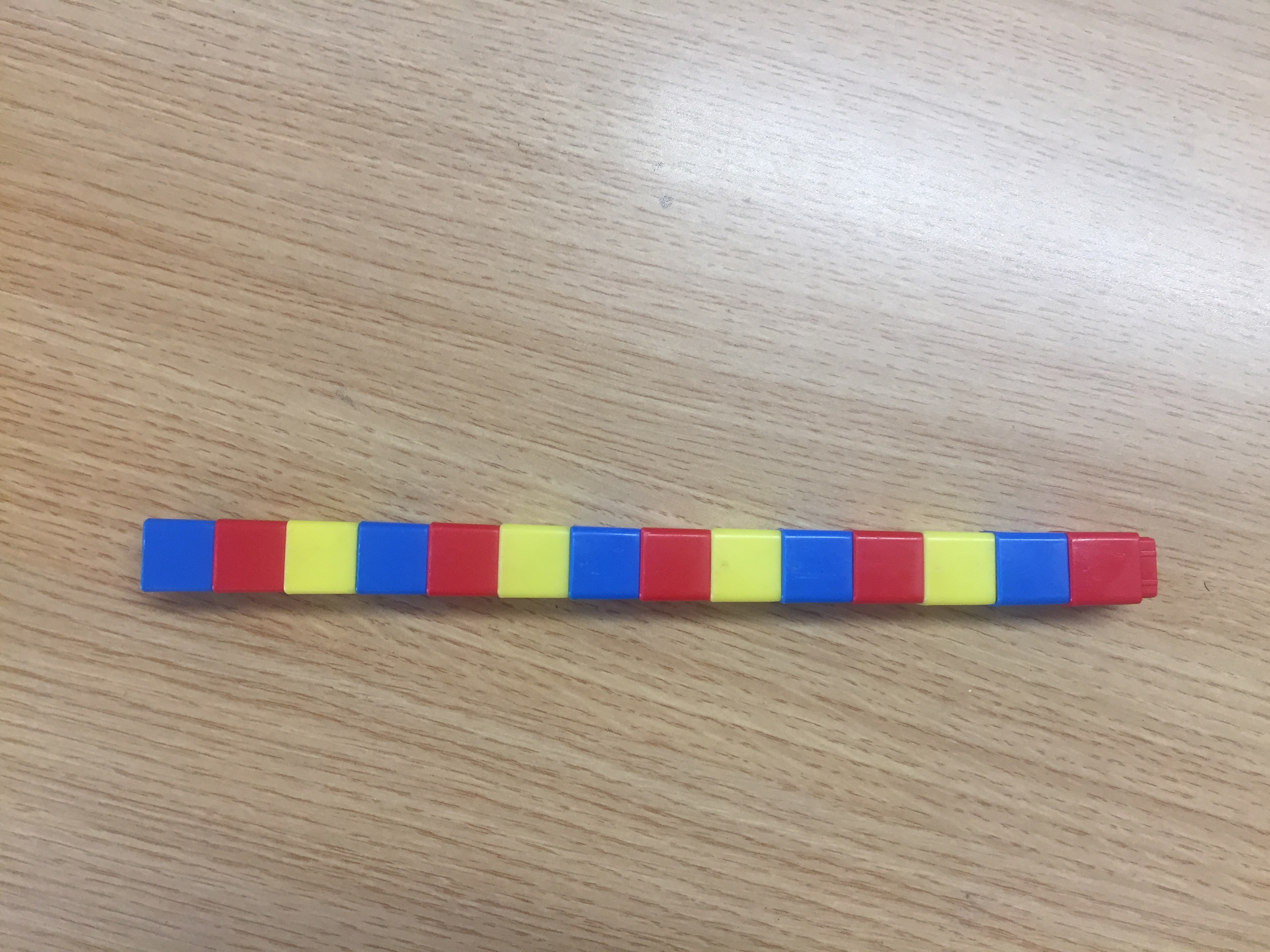Sequences

Unlike repeating patterns, sequences are more linear; they tend to increase or decrease in specific ways; thus they are also referred to as increasing and decreasing patterns. The way in which the terms (the individual parts of the sequence) are ordered is governed by a rule. Similar to repeating patterns, the children need to be able to identify this rule in order to extend the sequence. To help the children identify the rule in numerical sequences, they should be encouraged to examine each given term and identify what has happened between it and the next term i.e. did the numbers increase, decrease, by how much etc.? They should then record this (e.g. +2, –3) below and between the terms, as modelled in the Operation Maths Pupil books and Discovery books.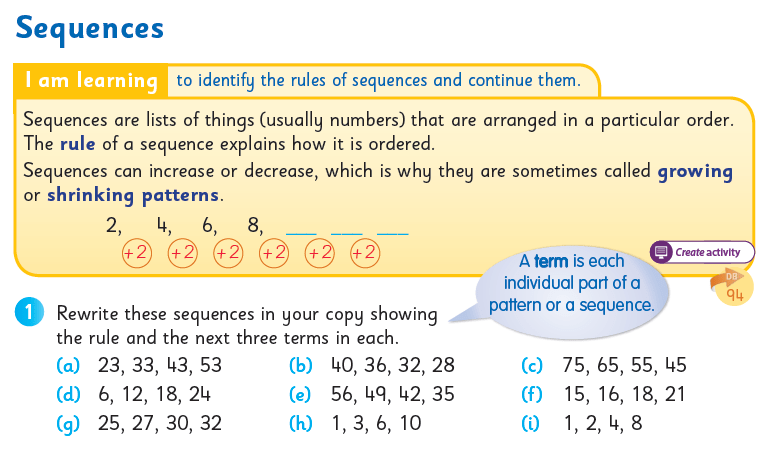Even when extending sequences in their copies, the children should be encouraged to leave an empty line below the sequences, to allow space for them to write in the differences between the terms.

Once the children are comfortable with sequences that increase/decrease by the same amount each term, they can progress to sequences that increase/decrease in varying but repetitive amounts, e.g. +2, +3, +2, …

Odd and even numbers

Odd and even numbers are an example of an increasing pattern/sequence, as the difference between each term is +2. Most children will find it simple enough to recognise odd and even numbers; typically they will tell you that if a number ends in 2, 4, 6, 8 or zero, is it even and, if it does not, it is odd. It is one thing to identify odd and even numbers in this way, but it’s another thing to visualise the numbers and appreciate why they are odd or even. Using concrete materials or pictorial representations is vital for the children to really develop their number sense
and their appreciation of how odd and even numbers interact.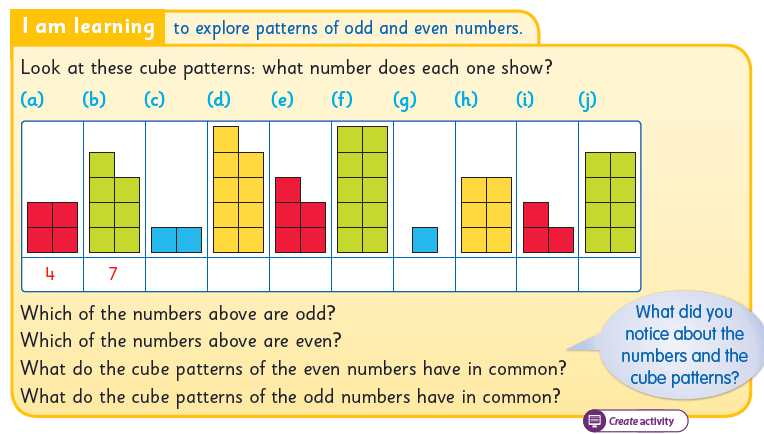As well as activities like that shown above, pairwise ten frames (ie ten frames placed vertically, as opposed to the more typical horizontal arrangement) can also be useful to model odd and even numbers. Such concrete or pictorial presentations can then be used to show how the total of an odd number and an odd number will always be even as the non-paired cube of both now join to form a pair. These activities also reinforce how only whole numbers can be classified as odd or even, even though a child may incorrectly assume that 1.2 is even, since it ends in 2.

Identifying patterns in addition and multiplication facts

Through concrete activities and activities using the 100 square, it is hoped that the children begin to appreciate the patterns in number facts and that that various groups of multiples are characterised by certain properties eg.:

• When adding/subtracting 10 on the 100 square the answer is always the number directly below/above the starting number.
• When adding/subtracting 9 on the 100 square the answer is always the number diagonally below left /diagonally above right the starting number.
• The multiples of 10 always end in zero
• The digits in the multiples of 9 always total 9 or a multiple of 9 (e.g. 9 × 11 = 99 and 9 + 9 = 18), etc.

Not only will knowledge like this greatly aid their ability to identify and recall the basic number facts, but it will also improve their ability to check and identify errors in their own, and others’ work (e.g. ‘173 × 5 = 858 … hmm, that can’t be correct because multiples of 5 should always end in 5 or 0; I need to do that again’).

Using T-charts to organise information

In the senior classes, when the rules governing the sequences are becoming more complex and less obvious, T-charts can be a very useful way to organise and present  the information. They can be particularly useful to help highlight the patterns and how these patterns are developing (see below). They can also provide the children with a clear way to explain how they see the rule. Therefore, the children should be encouraged to use them as a problem-solving strategy as much as is suitable.